Bayesian Analysis of a Linear Regression Model

Neter et al. (1996) describe a study of 54 patients undergoing a certain kind of liver operation in a surgical unit. The data set Surg contains survival time and certain covariates for each patient. Observations for the first 20 patients in the data set Surg are shown in Figure 39.7.

Figure 39.7 Surgical Unit Data
Obs x1 x2 x3 x4 y logy Logx1
1 6.7 62 81 2.59 200 2.3010 1.90211
2 5.1 59 66 1.70 101 2.0043 1.62924
3 7.4 57 83 2.16 204 2.3096 2.00148
4 6.5 73 41 2.01 101 2.0043 1.87180
5 7.8 65 115 4.30 509 2.7067 2.05412
6 5.8 38 72 1.42 80 1.9031 1.75786
7 5.7 46 63 1.91 80 1.9031 1.74047
8 3.7 68 81 2.57 127 2.1038 1.30833
9 6.0 67 93 2.50 202 2.3054 1.79176
10 3.7 76 94 2.40 203 2.3075 1.30833
11 6.3 84 83 4.13 329 2.5172 1.84055
12 6.7 51 43 1.86 65 1.8129 1.90211
13 5.8 96 114 3.95 830 2.9191 1.75786
14 5.8 83 88 3.95 330 2.5185 1.75786
15 7.7 62 67 3.40 168 2.2253 2.04122
16 7.4 74 68 2.40 217 2.3365 2.00148
17 6.0 85 28 2.98 87 1.9395 1.79176
18 3.7 51 41 1.55 34 1.5315 1.30833
19 7.3 68 74 3.56 215 2.3324 1.98787
20 5.6 57 87 3.02 172 2.2355 1.72277

Consider the model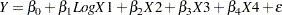where Y is the survival time, LogX1 is log(blood-clotting score), X2 is a prognostic index, X3 is an enzyme function test score, X4 is a liver function test score, andis an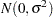error term.

A question of scientific interest is whether blood clotting score has a positive effect on survival time. Using PROC GENMOD, you can obtain a maximum likelihood estimate of the coefficient and construct a null point hypothesis to test whether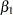is equal to 0. However, if you are interested in finding the probability that the coefficient is positive, Bayesian analysis offers a convenient alternative. You can use Bayesian analysis to directly estimate the conditional probability,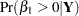, using the posterior distribution samples, which are produced as part of the output by PROC GENMOD.

The example that follows shows how to use PROC GENMOD to carry out a Bayesian analysis of the linear model with a normal error term. The SEED= option is specified to maintain reproducibility; no other options are specified in the BAYES statement. By default, a uniform prior distribution is assumed on the regression coefficients. The uniform prior is a flat prior on the real line with a distribution that reflects ignorance of the location of the parameter, placing equal likelihood on all possible values the regression coefficient can take. Using the uniform prior in the following example, you would expect the Bayesian estimates to resemble the classical results of maximizing the likelihood. If you can elicit an informative prior distribution for the regression coefficients, you should use the COEFFPRIOR= option to specify it. A default noninformative gamma prior is used for the scale parameter
.

You should make sure that the posterior distribution samples have achieved convergence before using them for Bayesian inference. PROC GENMOD produces three convergence diagnostics by default. If ODS Graphics is enabled as specified in the following SAS statements, diagnostic plots are also displayed. See the section Assessing Markov Chain Convergence for more information about convergence diagnostics and their interpretation.

Summary statistics of the posterior distribution samples are produced by default. However, these statistics might not be sufficient for carrying out your Bayesian inference, and further processing of the posterior samples might be necessary. The following SAS statements request the Bayesian analysis, and the OUTPOST= option saves the samples in the SAS data set PostSurg for further processing:

```ods graphics on;
proc genmod data=Surg;
model y = Logx1 X2 X3 X4 / dist=normal;
bayes seed=1 OutPost=PostSurg;
run;
ods graphics off;
```

The results of this analysis are shown in the following figures.

The "Model Information" table in Figure 39.8 summarizes information about the model you fit and the size of the simulation.

Figure 39.8 Model Information
The GENMOD Procedure

Bayesian Analysis

Model Information
Data Set WORK.SURG
Burn-In Size 2000
MC Sample Size 10000
Thinning 1
Sampling Algorithm Conjugate
Distribution Normal
Dependent Variable y Survival Time

The "Analysis of Maximum Likelihood Parameter Estimates" table in Figure 39.9 summarizes maximum likelihood estimates of the model parameters.

Figure 39.9 Maximum Likelihood Parameter Estimates
Analysis Of Maximum Likelihood Parameter Estimates
Parameter DF Estimate Standard Error Wald 95% Confidence Limits
Intercept 1 -730.559 85.4333 -898.005 -563.112
Logx1 1 171.8758 38.2250 96.9561 246.7954
x2 1 4.3019 0.5566 3.2109 5.3929
x3 1 4.0309 0.4996 3.0517 5.0100
x4 1 18.1377 12.0721 -5.5232 41.7986
Scale 1 59.8591 5.7599 49.5705 72.2832

 Note: The scale parameter was estimated by maximum likelihood.

Since no prior distributions for the regression coefficients were specified, the default noninformative uniform distributions shown in the "Uniform Prior for Regression Coefficients" table in Figure 39.10 are used. Noninformative priors are appropriate if you have no prior knowledge of the likely range of values of the parameters, and if you want to make probability statements about the parameters or functions of the parameters. See, for example, Ibrahim, Chen, and Sinha (2001) for more information about choosing prior distributions.

Figure 39.10 Regression Coefficient Priors
The GENMOD Procedure

Bayesian Analysis

Uniform Prior for Regression
Coefficients
Parameter Prior
Intercept Constant
Logx1 Constant
x2 Constant
x3 Constant
x4 Constant

The default noninformative gamma prior distribution for the normal scale parameter is shown in the "Independent Prior Distributions for Model Parameters" table in Figure 39.11.

Figure 39.11 Scale Parameter Prior
Independent Prior Distributions for Model Parameters
Parameter Prior Distribution Hyperparameters
Shape Scale
Dispersion Inverse Gamma 2.001 0.0001

By default, the maximum likelihood estimates of the regression parameters are used as the starting values for the simulation when noninformative prior distributions are used. These are listed in the "Initial Values and Seeds" table in Figure 39.12.

Figure 39.12 MCMC Initial Values and Seeds
Initial Values of the Chain
Chain Seed Intercept Logx1 x2 x3 x4 Dispersion
1 1 -730.559 171.8758 4.301896 4.030878 18.1377 3223.694

Summary statistics for the posterior sample are displayed in the "Fit Statistics," "Descriptive Statistics for the Posterior Sample," "Interval Statistics for the Posterior Sample," and "Posterior Correlation Matrix" tables in Figure 39.13, Figure 39.14, Figure 39.15, and Figure 39.16, respectively.

Figure 39.13 Fit Statistics
Fit Statistics
DIC (smaller is better) 608.411
pD (effective number of parameters) 6.571

Figure 39.14 Descriptive Statistics
The GENMOD Procedure

Bayesian Analysis

Posterior Summaries
Parameter N Mean Standard
Deviation
Percentiles
25% 50% 75%
Intercept 10000 -730.1 91.0133 -789.6 -729.6 -670.5
Logx1 10000 171.7 40.3792 144.3 171.8 198.6
x2 10000 4.3000 0.5989 3.8990 4.2932 4.6951
x3 10000 4.0310 0.5354 3.6645 4.0265 4.3910
x4 10000 18.0888 12.8949 9.4919 18.0430 26.7881
Dispersion 10000 3795.9 770.4 3247.6 3694.7 4238.2

Figure 39.15 Interval Statistics
Posterior Intervals
Parameter Alpha Equal-Tail Interval HPD Interval
Intercept 0.050 -908.7 -551.0 -906.2 -549.2
Logx1 0.050 92.4773 251.6 94.2813 253.0
x2 0.050 3.1062 5.4839 3.1747 5.5328
x3 0.050 2.9812 5.1041 2.9532 5.0612
x4 0.050 -7.2646 43.6506 -5.9839 44.6427
Dispersion 0.050 2569.0 5548.5 2389.4 5308.8

Figure 39.16 Posterior Sample Correlation Matrix
Posterior Correlation Matrix
Parameter Intercept Logx1 x2 x3 x4 Dispersion
Intercept 1.000 -0.856 -0.580 -0.712 0.579 -0.002
Logx1 -0.856 1.000 0.285 0.490 -0.636 0.009
x2 -0.580 0.285 1.000 0.302 -0.492 -0.007
x3 -0.712 0.490 0.302 1.000 -0.616 -0.004
x4 0.579 -0.636 -0.492 -0.616 1.000 0.002
Dispersion -0.002 0.009 -0.007 -0.004 0.002 1.000

Since noninformative prior distributions were used, the posterior sample means, standard deviations, and interval statistics shown in Figure 39.13 and Figure 39.14 are consistent with the maximum likelihood estimates shown in Figure 39.9.

By default, PROC GENMOD computes three convergence diagnostics: the lag1, lag5, lag10, and lag50 autocorrelations (Figure 39.17); Geweke diagnostic statistics (Figure 39.18); and effective sample sizes (Figure 39.19). There is no indication that the Markov chain has not converged. See the section Assessing Markov Chain Convergence for more information about convergence diagnostics and their interpretation.

Figure 39.17 Posterior Sample Autocorrelations
The GENMOD Procedure

Bayesian Analysis

Posterior Autocorrelations
Parameter Lag 1 Lag 5 Lag 10 Lag 50
Intercept -0.0050 -0.0023 -0.0138 0.0032
Logx1 0.0030 -0.0063 -0.0070 -0.0034
x2 -0.0113 -0.0046 -0.0235 -0.0139
x3 0.0019 0.0064 -0.0073 0.0047
x4 -0.0001 -0.0084 0.0050 -0.0084
Dispersion -0.0019 0.0088 -0.0297 0.0025

Figure 39.18 Geweke Diagnostic Statistics
Geweke Diagnostics
Parameter z Pr > |z|
Intercept -0.8783 0.3798
Logx1 1.4800 0.1389
x2 -0.0438 0.9651
x3 0.1000 0.9204
x4 -0.8893 0.3739
Dispersion 0.1011 0.9195

Figure 39.19 Effective Sample Sizes
Effective Sample Sizes
Parameter ESS Autocorrelation
Time
Efficiency
Intercept 10000.0 1.0000 1.0000
Logx1 10000.0 1.0000 1.0000
x2 10232.2 0.9773 1.0232
x3 10000.0 1.0000 1.0000
x4 10000.0 1.0000 1.0000
Dispersion 10000.0 1.0000 1.0000

Trace, autocorrelation, and density plots for the seven model parameters, shown in Figure 39.20 through Figure 39.25, are useful in diagnosing whether the Markov chain of posterior samples has converged. These plots show no evidence that the chain has not converged. See the section Visual Analysis via Trace Plots for help with interpreting these diagnostic plots.

Figure 39.20 Diagnostic Plots for Intercept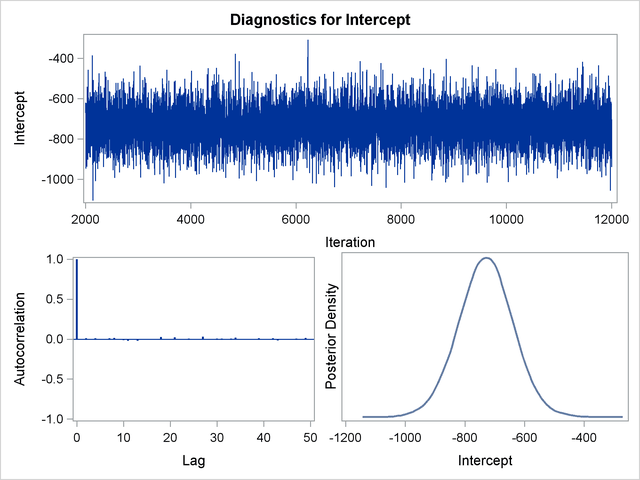Figure 39.21 Diagnostic Plots for logX1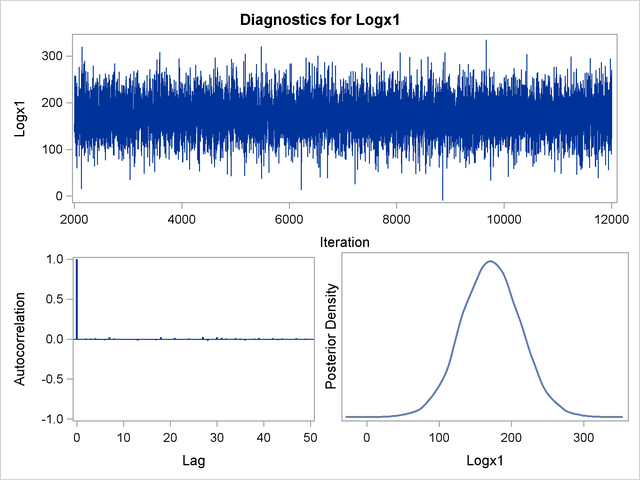Figure 39.22 Diagnostic Plots for X2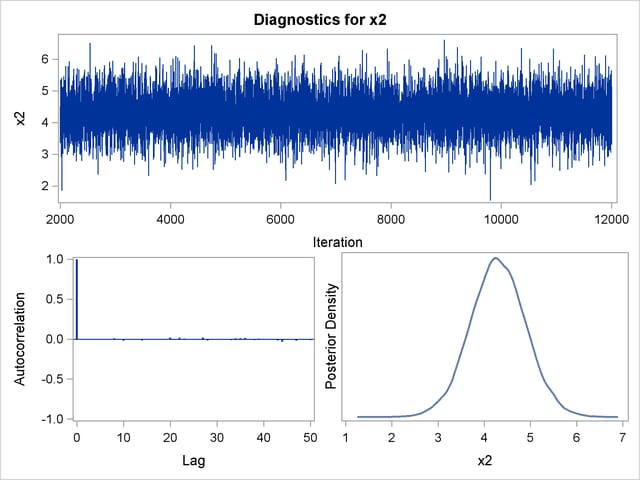Figure 39.23 Diagnostic Plots for X3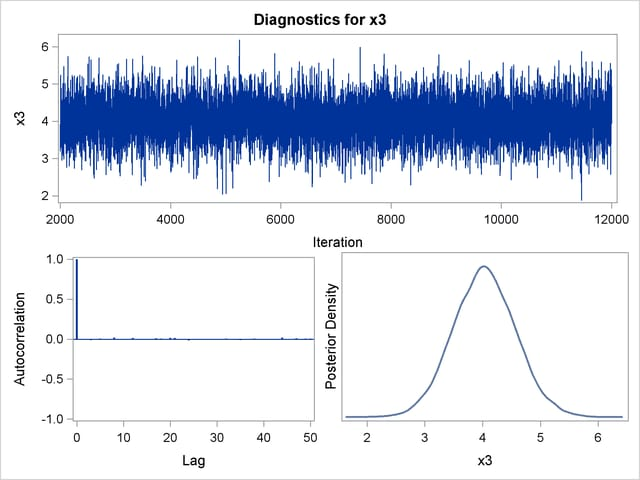Figure 39.24 Diagnostic Plots for X4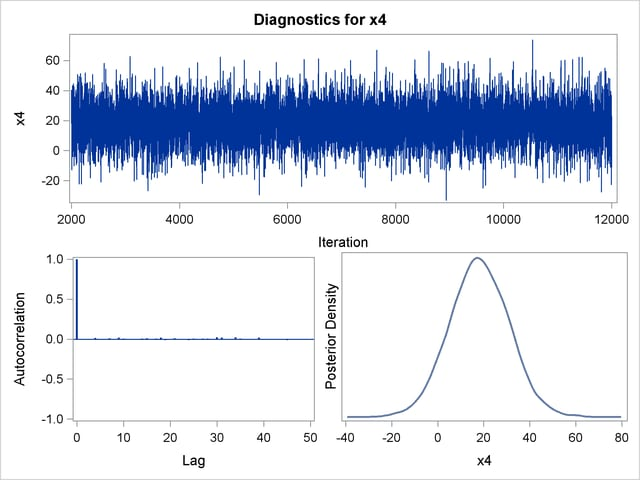Figure 39.25 Diagnostic Plots for X5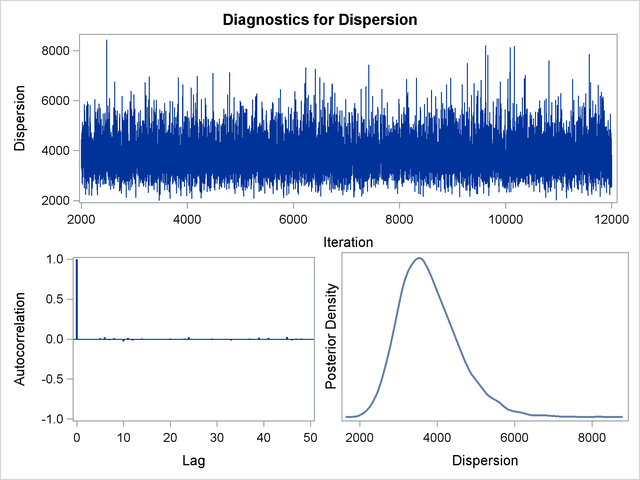Suppose, for illustration, a question of scientific interest is whether blood clotting score has a positive effect on survival time. Since the model parameters are regarded as random quantities in a Bayesian analysis, you can answer this question by estimating the conditional probability ofbeing positive, given the data,, from the posterior distribution samples. The following SAS statements compute the estimate of the probability ofbeing positive:

```data Prob;
set PostSurg;
Indicator = (logX1 > 0);
label Indicator= 'log(Blood Clotting Score) > 0';
run;

proc Means data = Prob(keep=Indicator) n mean;
run;
```

As shown in Figure 39.26, there is a 1.00 probability of a positive relationship between the logarithm of a blood clotting score and survival time, adjusted for the other covariates.

Figure 39.26 Probability That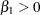The MEANS Procedure

Analysis Variable : Indicator
log(Blood Clotting Score)
> 0
N Mean
10000 0.9999000